## introduction

latticeExtra is an R package (i.e. a package for the R statistical computing environment), providing functions for generating statistical graphics. It extends the Lattice framework (lattice package), which is an implementation of Trellis graphics in R.

Many of the functions and datasets in latticeExtra are described in Deepayan Sarkar's book, Lattice: Multivariate Data Visualization with R. The book web site also includes figures and code.

This site serves as a reference for the R functions and datasets in the package. The help pages for all functions are also available as a PDF document -- the "Reference manual" -- from any CRAN mirror (see link below).

The package is maintained by Deepayan Sarkar and Felix Andrews.

## index

 ancestry Modal ancestry by County according to US 2000 Census as.layer Overlay panels of Trellis plots on same or different scales biocAccess Hourly access attempts to Bioconductor website combineLimits Combine axis limits across margins c.trellis Merge trellis objects, using same or different scales custom.theme Create a lattice theme based on specified colors dendrogramGrob Create a Grob Representing a Dendrogram doubleYScale Draw two plot series with different y scales EastAuClimate Climate of the East Coast of Australia ecdfplot Trellis Displays of Empirical CDF ggplot2like A ggplot2-like theme for Lattice gvhd10 Flow cytometry data from five samples from a patient horizonplot Plot many time series in parallel layer Add layers to a lattice plot, optionally using a new data source mapplot Trellis displays on Maps a.k.a. Choropleth maps marginal.plot Display marginal distributions panel.2dsmoother Plot a smooth approximation of z over x and y. panel.3dmisc Miscellanous panel utilities for three dimensional Trellis Displays panel.ablineq Draw a line with a label, by default its equation panel.ellipse Lattice panel function to fit and draw a confidence ellipsoid from bivariate data. panel.key Draw a simple key inside a panel of a lattice plot. panel.levelplot.points Panel functions for level-coded irregular points panel.quantile Plot a quantile regression line with standard error bounds. panel.scaleArrow Draw a scale bar as an arrow, labelled with its length in plot units. panel.smoother Plot a smoothing line with standard error bounds. panel.tskernel Calculate and plot smoothed time series. panel.xblocks Plot contiguous blocks along x axis. panel.xyarea Plot series as filled polygons. postdoc Reasons for Taking First Postdoctoral Appointment resizePanels Resize panels to match data scales rootogram Trellis Displays of Tukey's Hanging Rootograms scale.components Custom lattice axis scales SeatacWeather Daily Rainfall and Temperature at the Seattle-Tacoma Airport segplot Plot segments using the Trellis framework style example This is a sample plot to demonstrate different graphical settings (themes). theEconomist.theme Generate plots with style like The Economist. tileplot Plot a spatial mosaic from irregular 2D points USAge US national population estimates USCancerRates Rate of Death Due to Cancer in US Counties useOuterStrips Put Strips on the Boundary of a Lattice Display xyplot.stl Display stl fits with Lattice

# general statistical plots

## rootogram

Trellis Displays of Tukey's Hanging Rootograms

One example:```rootogram(~x, dfun = function(x) dpois(x, lambda = 50))
```

Source code

## segplot

Plot segments using the Trellis framework

One example: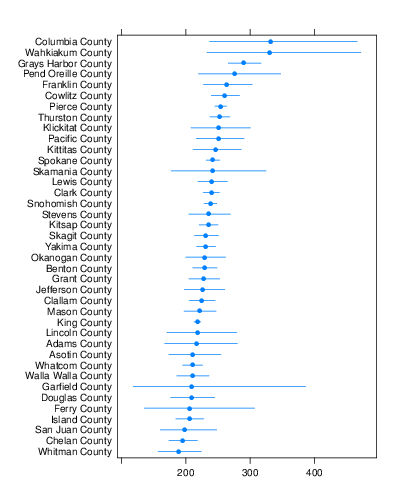```segplot(reorder(factor(county), rate.male) ~ LCL95.male +
UCL95.male, data = subset(USCancerRates, state ==
"Washington"), draw.bands = FALSE, centers = rate.male)
```

Source code

## ecdfplot

Trellis Displays of Empirical CDF

One example:```ecdfplot(~height | voice.part, data = singer)
```

Source code

## marginal.plot

Display marginal distributions

One example: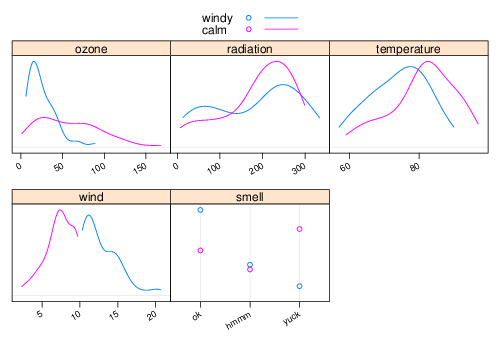```marginal.plot(enviro[, 1:5], data = enviro, groups = is.windy,
auto.key = list(lines = TRUE))
```

Source code

# functions of one variable

## xyplot.stl

Display stl fits with Lattice

One example: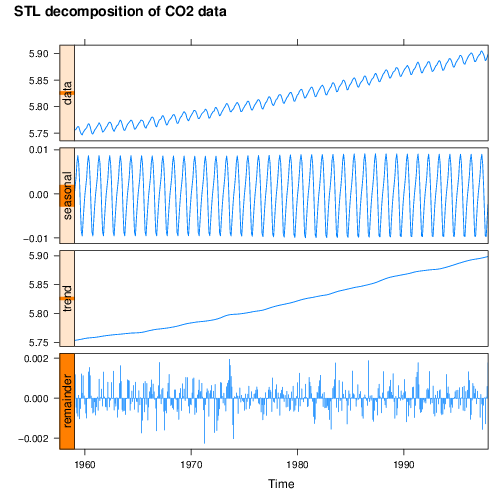```xyplot(stl(log(co2), s.window = 21), main = "STL decomposition of CO2 data")
```

Source code

## panel.smoother

Plot a smoothing line with standard error bounds.

One example:```xyplot(y + y2 ~ x, xy) + glayer(panel.smoother(...))
```

Source code

## panel.quantile

Plot a quantile regression line with standard error bounds.

One example:```update(xyplot(y ~ x, xy) + layer(panel.quantile(x,
y, tau = c(0.5, 0.9, 0.1), superpose = TRUE)),
auto.key = list(text = paste(c(50, 90, 10), "% quantile"),
points = FALSE, lines = TRUE))
```

Source code

## panel.xblocks

Plot contiguous blocks along x axis.

One example:```xyplot(flow ~ flowdates, type = "l") + layer_(panel.xblocks(x,
months, col = c("lightgray", "#e6e6e6"), border = "darkgray"))
```

Source code

## panel.xyarea

Plot series as filled polygons.

One example:```xyplot(ts.union(data = sunspot.year, lag10 = lag(sunspot.year,
10)), aspect = "xy", cut = list(n = 3, overlap = 0),
superpose = TRUE, panel = panel.superpose, panel.groups = function(...,
group.number) {
if (group.number ==....
```

Source code

## panel.tskernel

Calculate and plot smoothed time series.

One example: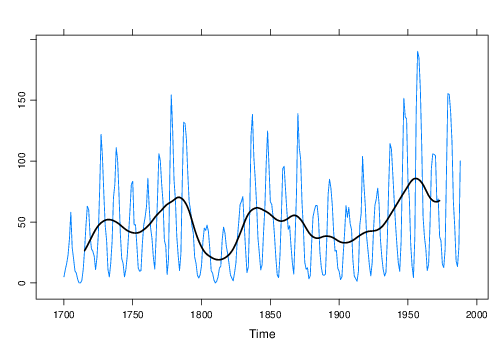```xyplot(sunspot.year) + layer(panel.tskernel(x, y,
width = 20, c = 3, col = 1, lwd = 2))
```

Source code

## horizonplot

Plot many time series in parallel

One example:```horizonplot(dat, horizonscale = 10, colorkey = TRUE,
layout = c(1, 12), strip.left = FALSE, ylab = list(rev(colnames(dat)),
rot = 0, cex = 0.7)) + layer_(panel.fill(col = "gray90"),
panel.xblocks(..., col = "white"))
```

Source code

# functions of two variables

## mapplot

Trellis displays on Maps a.k.a. Choropleth maps

One example:```mapplot(county ~ log10(population), ancestry, map = county.map,
colramp = NULL)
```

Source code

## tileplot

Plot a spatial mosaic from irregular 2D points

One example:```tileplot(z ~ x * y, xyz, border = "black", col.regions = grey.colors(100),
pch = ifelse(is.na(xyz\$z), 4, 21), panel = function(...) {
panel.fill("hotpink")
panel.voronoi(...)
})
```

Source code

## panel.levelplot.points

Panel functions for level-coded irregular points

One example:```levelplot(depth ~ long + lat | Magnitude, data = quakes.ordered,
panel = panel.levelplot.points, type = c("p",
"g"), aspect = "iso", prepanel = prepanel.default.xyplot)
```

Source code

## panel.2dsmoother

Plot a smooth approximation of z over x and y.

One example:```levelplot(z ~ x * y, xyz, panel = panel.levelplot.points,
cex = 1.2) + layer_(panel.2dsmoother(..., n = 200))
```

Source code

## panel.ellipse

Lattice panel function to fit and draw a confidence ellipsoid from bivariate data.

One example:```xyplot(Sepal.Length ~ Petal.Length, groups = Species,
data = iris, scales = "free", par.settings = list(superpose.symbol = list(pch = c(15:17)),
superpose.line = list(lwd = 2, lty = 1:3)),
panel = function(x, y, ...) {
pane....
```

Source code

## dendrogramGrob

Create a Grob Representing a Dendrogram

One example:```levelplot(x[row.ord, col.ord], aspect = "fill", scales = list(x = list(rot = 90)),
colorkey = list(space = "left"), legend = list(right = list(fun = dendrogramGrob,
args = list(x = dd.col, ord = col.ord, side = "right",
si....
```

Source code

# utilities

## useOuterStrips

Put Strips on the Boundary of a Lattice Display

One example: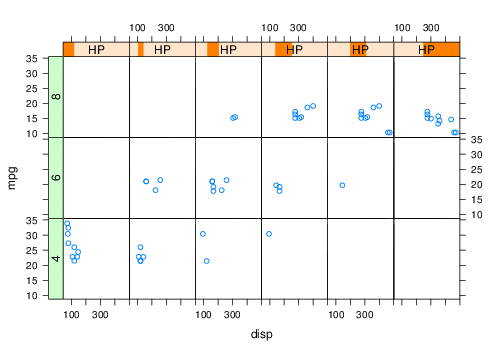```xyplot(mpg ~ disp | HP + factor(cyl), mtcars, layout = dimx,
par.strip.text = list(lines = 0.5), strip = new.strip,
strip.left = new.strip.left, par.settings = par.settings)
```

Source code

## resizePanels

Resize panels to match data scales

One example:```dotplot(state.name ~ Frost | reorder(state.region,
Frost), data = state, layout = c(1, 4), scales = list(y = list(relation = "free")),
par.settings = list(layout.heights = list(panel = h),
layout.widths = list(panel = w)))
```

Source code

## combineLimits

Combine axis limits across margins

One example:```xyplot(Price ~ EngineSize | reorder(AirBags, Price) +
Cylinders, data = Cars93, subset = Cylinders !=
"rotary" & Cylinders != "5", scales = list(relation = "free",
y = list(log = FALSE, tick.number = 3, rot = 0)),
xlab = "Engine S....
```

Source code

## scale.components

Custom lattice axis scales

One example:```xyplot((1:200)/20 ~ (1:200)/20, type = c("p", "g"),
scales = list(x = list(log = 2), y = list(log = 10)),
xscale.components = xscale.components.fractions,
yscale.components = yscale.components.log10ticks)
```

Source code

## panel.ablineq

Draw a line with a label, by default its equation

One example:```update(xyplot(ysim ~ xsim) + layer(panel.ablineq(lm(y ~
x), r.sq = TRUE, rot = TRUE, at = 0.8, pos = 3),
style = 1) + layer(panel.ablineq(lm(y ~ x + 0),
r.sq = TRUE, rot = TRUE, at = 0.8, pos = 1),
style = 2), auto.key = list(text....
```

Source code

## panel.scaleArrow

Draw a scale bar as an arrow, labelled with its length in plot units.

One example:```xyplot(EuStockMarkets) + layer(panel.scaleArrow(x = 0.99,
append = " units", col = "grey", srt = 90, cex = 0.8))
```

Source code

## panel.3dmisc

Miscellanous panel utilities for three dimensional Trellis Displays

One example:```cloud(VADeaths, panel.3d.cloud = panel.3dbars, xbase = 0.4,
ybase = 0.4, zlim = c(0, max(VADeaths)), scales = list(arrows = FALSE,
just = "right"), xlab = NULL, ylab = NULL,
col.facet = level.colors(VADeaths, at = do.breaks(range(....
```

Source code

## panel.key

Draw a simple key inside a panel of a lattice plot.

One example:```xyplot(ozone ~ wind | equal.count(temperature, 2),
data = environmental) + layer(panel.loess(x,
y, span = 0.5), style = 1) + layer(panel.loess(x,
y, span = 1), style = 2) + layer(panel.key(c("span = 0.5",
"span = 1.0"), corner = c....
```

Source code

# extended trellis framework

## layer

Add layers to a lattice plot, optionally using a new data source

One example:```qua + layer_(panel.contourplot(x = gridlon, y = gridlat,
z = dist, contour = TRUE, subscripts = TRUE),
data = newdat)
```

Source code

## as.layer

Overlay panels of Trellis plots on same or different scales

One example:```xyplot(fdeaths ~ mdeaths) + as.layer(bwplot(~mdeaths,
box.ratio = 0.2), y.same = FALSE)
```

Source code

## doubleYScale

Draw two plot series with different y scales

One example: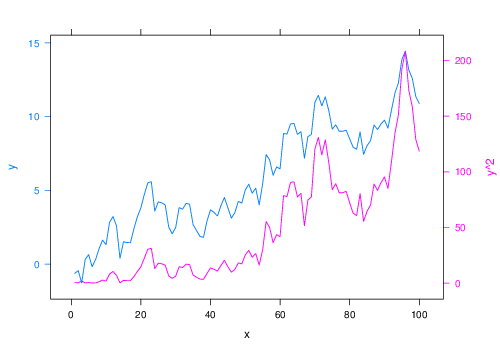```doubleYScale(obj1 = obj1, obj2 = obj2, add.ylab2 = TRUE)
```

Source code

## c.trellis

Merge trellis objects, using same or different scales

One example:```update(c(xyplot(lat ~ long | equal.count(depth, 3),
quakes, aspect = "iso", pch = ".", cex = 2, xlab = NULL,
ylab = NULL), histogram(quakes\$depth), x.same = FALSE,
y.same = FALSE, layout = c(4, 1)), scales = list(at = list(NULL,
N....
```

Source code

# styles

## style example

This is a sample plot to demonstrate different graphical settings (themes).

One example:```xyplot(Sepal.Length + Sepal.Width ~ Petal.Length +
Petal.Width, iris, type = c("p", "r"), jitter.x = TRUE,
jitter.y = TRUE, factor = 5, auto.key = list(lines = TRUE,
rectangles = TRUE), legend = list(right = list(fun = "draw.color....
```

## custom.theme

Create a lattice theme based on specified colors

One example:```xyplot(Sepal.Length + Sepal.Width ~ Petal.Length +
Petal.Width, iris, type = c("p", "r"), jitter.x = TRUE,
jitter.y = TRUE, factor = 5, auto.key = list(lines = TRUE,
rectangles = TRUE), legend = list(right = list(fun = "draw.color....
```

Source code

## ggplot2like

A ggplot2-like theme for Lattice

One example: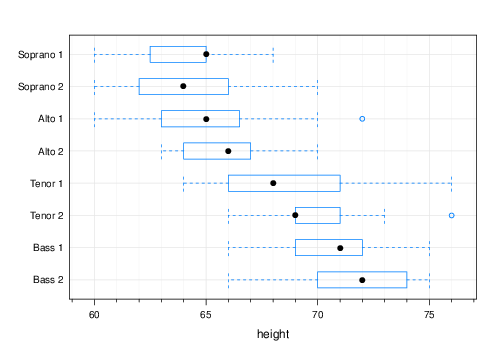```bwplot(voice.part ~ height, data = singer)
```

Source code

## theEconomist.theme

Generate plots with style like The Economist.

One example:```trellis.last.object() + layer_(panel.xblocks(x, x >=
1980, col = "#6CCFF6", alpha = 0.5)) + layer(panel.text(1988,
180, "Forecast", font = 3, pos = 2))
```

Source code

# data

## USAge

US national population estimates

One example:```xyplot(Population ~ Age | factor(Year), USAge.df,
groups = Sex, type = c("l", "g"), auto.key = list(points = FALSE,
lines = TRUE, columns = 2), aspect = "xy",
ylab = "Population (millions)", subset = Year %in%
seq(1905, 19....
```

## USCancerRates

Rate of Death Due to Cancer in US Counties

One example:```mapplot(rownames(USCancerRates) ~ log(rate.male) +
log(rate.female), data = USCancerRates, map = map("county",
plot = FALSE, fill = TRUE, projection = "mercator"))
```

## ancestry

Modal ancestry by County according to US 2000 Census

## postdoc

Reasons for Taking First Postdoctoral Appointment

One example:```barchart(prop.table(postdoc, margin = 1), auto.key = TRUE,
xlab = "Proportion")
```

## biocAccess

Hourly access attempts to Bioconductor website

One example:```xyplot(stl(ts(biocAccess\$counts[1:(24 * 30)], frequency = 24),
"periodic"))
```

## gvhd10

Flow cytometry data from five samples from a patient

One example:```histogram(~log2(FSC.H) | Days, gvhd10, xlab = "log Forward Scatter",
type = "density", nint = 50, layout = c(2, 4))
```

## SeatacWeather

Daily Rainfall and Temperature at the Seattle-Tacoma Airport

One example: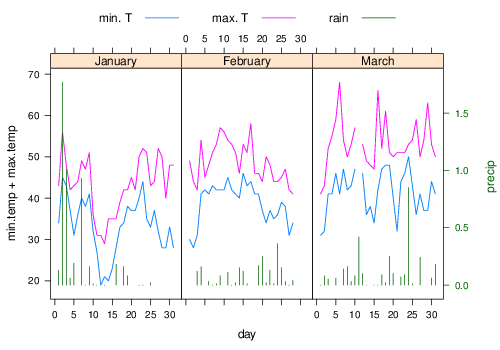```doubleYScale(obj1 = temp, obj2 = rain, style1 = 0,
style2 = 3, add.ylab2 = TRUE, text = c("min. T",
"max. T", "rain"), columns = 3)
```

## EastAuClimate

Climate of the East Coast of Australia

One example:```segplot(Latitude ~ WinterMinTemp + SummerMaxTemp,
EastAuClimate, main = "Average daily temperature ranges n of coastal Australian sites",
ylab = "Latitude", xlab = "Temperature (degrees C)",
par.settings = simpleTheme(lwd = 3, alpha ....
```
latticeExtra version 0.6-25 on R version 3.0.1 (at 2013-09-25)

fonts by exljbris
web dev by felix andrews